##### The Japanese Bonsai specialist
Direct order Contact Help / Services Newsletter# Ledebouria cooperi (cooper's false scilla)

Lederbouria cooperi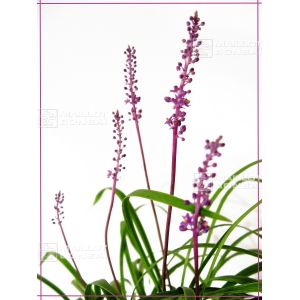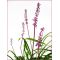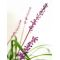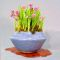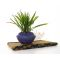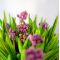ref. : 82425,00

This item is temporarily unavailableNotify availability

###### Description

Vivacious plant, rhizomes. Sold with a plastic pot. 50 mm wide x 70 mm high.

Ledebouria cooperi is popular with amateur kusamono gardeners due to its small size and purple flowers that bloom in spring. It flowers from March to April and afterwards develops tough green leaves decorated with stripes of brown-black. The foliage disappears completely in winter.

Origin: South Africa

Flower Colour: Purple

Preferred Conditions: Well drained soil

Preferred Aspect: Sun or mid shade

#ledebouria 4 #kusamono 3.8 #cooperi 3.7 #small 3.5 #with 3.4 #preferred 2.9 #flowers 2.7 #plants 2.6 #cooper 2.6 #purple 2.6

Formule
(( ROUND((CHAR_LENGTH(b.article_nom)-CHAR_LENGTH(REPLACE(b.article_nom, 'ledebouria', '')))/LENGTH('ledebouria')) + ROUND((CHAR_LENGTH(b.article_description)-CHAR_LENGTH(REPLACE(b.article_description, 'ledebouria', '')))/LENGTH('ledebouria')) ) * 4) + (( ROUND((CHAR_LENGTH(b.article_nom)-CHAR_LENGTH(REPLACE(b.article_nom, 'cooperi', '')))/LENGTH('cooperi')) + ROUND((CHAR_LENGTH(b.article_description)-CHAR_LENGTH(REPLACE(b.article_description, 'cooperi', '')))/LENGTH('cooperi')) ) * 3.7) + (( ROUND((CHAR_LENGTH(b.article_nom)-CHAR_LENGTH(REPLACE(b.article_nom, 'with', '')))/LENGTH('with')) + ROUND((CHAR_LENGTH(b.article_description)-CHAR_LENGTH(REPLACE(b.article_description, 'with', '')))/LENGTH('with')) ) * 3.4) + (( ROUND((CHAR_LENGTH(b.article_nom)-CHAR_LENGTH(REPLACE(b.article_nom, 'preferred', '')))/LENGTH('preferred')) + ROUND((CHAR_LENGTH(b.article_description)-CHAR_LENGTH(REPLACE(b.article_description, 'preferred', '')))/LENGTH('preferred')) ) * 2.9) + (( ROUND((CHAR_LENGTH(b.article_nom)-CHAR_LENGTH(REPLACE(b.article_nom, 'flowers', '')))/LENGTH('flowers')) + ROUND((CHAR_LENGTH(b.article_description)-CHAR_LENGTH(REPLACE(b.article_description, 'flowers', '')))/LENGTH('flowers')) ) * 2.7) + (( ROUND((CHAR_LENGTH(b.article_nom)-CHAR_LENGTH(REPLACE(b.article_nom, 'cooper', '')))/LENGTH('cooper')) + ROUND((CHAR_LENGTH(b.article_description)-CHAR_LENGTH(REPLACE(b.article_description, 'cooper', '')))/LENGTH('cooper')) ) * 2.6) + (( ROUND((CHAR_LENGTH(b.article_nom)-CHAR_LENGTH(REPLACE(b.article_nom, 'scilla', '')))/LENGTH('scilla')) + ROUND((CHAR_LENGTH(b.article_description)-CHAR_LENGTH(REPLACE(b.article_description, 'scilla', '')))/LENGTH('scilla')) ) * 2.6) + (( ROUND((CHAR_LENGTH(b.article_nom)-CHAR_LENGTH(REPLACE(b.article_nom, 'purple', '')))/LENGTH('purple')) + ROUND((CHAR_LENGTH(b.article_description)-CHAR_LENGTH(REPLACE(b.article_description, 'purple', '')))/LENGTH('purple')) ) * 2.6) + (( ROUND((CHAR_LENGTH(b.article_nom)-CHAR_LENGTH(REPLACE(b.article_nom, 'false', '')))/LENGTH('false')) + ROUND((CHAR_LENGTH(b.article_description)-CHAR_LENGTH(REPLACE(b.article_description, 'false', '')))/LENGTH('false')) ) * 2.5) + (( ROUND((CHAR_LENGTH(b.article_nom)-CHAR_LENGTH(REPLACE(b.article_nom, 'disappears', '')))/LENGTH('disappears')) + ROUND((CHAR_LENGTH(b.article_description)-CHAR_LENGTH(REPLACE(b.article_description, 'disappears', '')))/LENGTH('disappears')) ) * 2)

## Secure payment## Delivery

Our logistic partners :04 74 55 23 48
Pépinière MAILLOT-BONSAÏ
Le Bois Frazy
01990 RELEVANT - FRANCE
on appointment